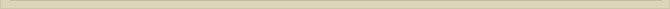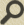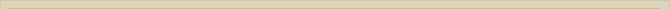## The Lorentzfactor and the ether

When you are interested in physics you must read “Unbelievable“!

The ether must be consistent with observations. The assumed ether must describe the relativistic phenomena in an abstract, mathematical way. The disadvantage of describing physics only in mathematical formulas is that the mathematical abstraction diminishes the perception of physical processes to the mathematical outcome. The ether theory on the contrary offers possibilities, apart from mathematical formulation, to describe the physical process through which new insights are achieved.

In high-energy physics elementary particles like electrons and protons have experimentally a speed limit c. The observation that particles cannot be accelerated above the speed of light, is seen as an empirical proof of the SRT. This conclusion is however premature when there is ether. With ether the speed of light is the consequence of the inert qualities of ether. If the speed of adjustment of electromagnetic changes in the ether is limited to c, it is not so hard to comprehend that electromagnetic particles cannot be accelerated beyond the electromagnetic speed c, when the ether itself adjust with c.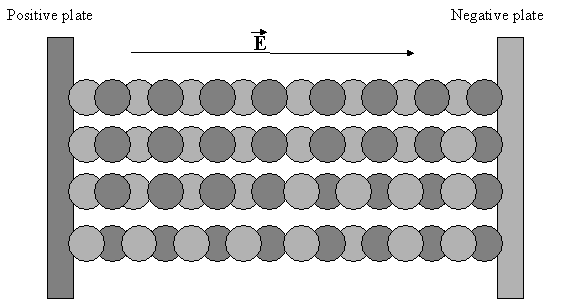Figure 9.  The charge division in ether between condenser plates.

The dielectric movement and the electric field in the ether are schematically illustrated in figure 9. The overlapping parts of the circles represent only figurative the dielectric movement in the point-volumes between the positive and negative plates of a condenser. The charges of the plates are compensated the charge displacement in the point-volumes; the ether is in equilibrium.

A particle without charge will, when placed in an electric field, not be subdued to a force. When however a charged particle enters the field between the plates the electric field of the particle and that of the condenser will interact. The resulting electric field will be a vector summation of both fields, as science describes. We assume that a charged particle +Q enters the field in the condenser. The force in an electric field on a charged particle is realized the ether itself. The charged plates draw and push on the particle means of the ether as the medium.

The polarized ether around a charged particle, the induced electric field in the ether  its own charge, is symmetric around the particle. The polarized ether around the single charge +Q is symmetric and therefore induces no resulting force on the particle.  When however this charged particle is placed in an external electric field this symmetry is lost. The force on the particle +Q is no longer equal in all directions. The resulting force is proportional to the resulting field; the vector summation of both fields.

F=Q.E

The dielectric displacement around charge Q combined with the external electric field in the ether between the condenser plates will result in an asymmetric electric field surrounding Q.  The resulting force F will be proportional and in the direction of the external field E.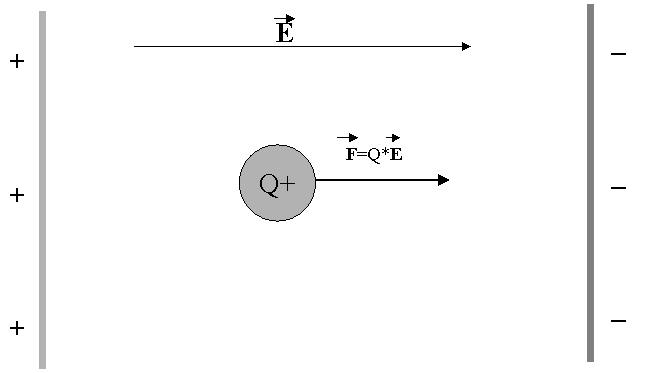Figure 10.   The force on a charged particle +Q in an electric field and ether.

The force F will result in an acceleration of particle Q in the direction of E.

The speed v of Q, combined with the inert properties of a vacuum given  c, will be responsible for the fact that not all the ether around Q can adjust in time to the changed conditions. The effect of this inert quality of vacuum is presented in figure 11. Particle Q is presented in figure 11  a black spot. During the small time interval dt particle Q moves a distance vdt. The field around Q, presented a sphere with radius cdt, will adjust to the change of position of Q in dt seconds. The ability of the ether to adjust in dt seconds is limited to the space around Q with a radius of cdt. Because of the deterministic law that reaction can only take place after action, the ether can adjust to the changed circumstances only after Q moved vdt meter. The inert property of the ether is thus responsible for the fact that the adjustment of the ether to the new situation, where Q moved vdt meter, will take place after the change of position of Q.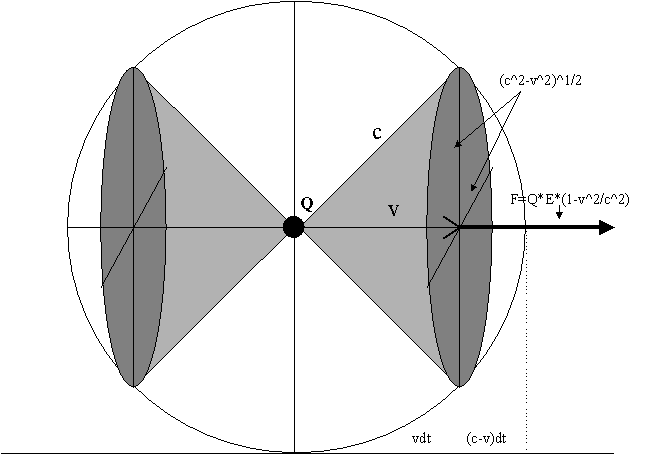Figure 11.   The decline of the electrostatic force in vacuum caused motion.

In the next dt seconds the ether can adjust. The adjustment of the ether will always lag the primal change. After Q moved vdt meters the ether can adjust with speed c. The ether between the original position of Q and vdt cannot be adjusted in time because Q already passed. Only the ether around the original position of Q, between vdt and cdt, is adjusted in time to contribute to the force accelerating Q in the next dt seconds.

The resulting force on Q is therefore realized a fraction of the polarized ether. The radius of the circle at vdt passing the acceleration force on to Q, with speed v, is a factor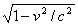of the original circle (R=cdt). This is the Lorentz-factor, which will be discussed further in the chapter the “The Photon”.

The force on the particle in the ether is however not realized a line, but the surface of the ether still contributing to the force. The surface of the ether still able to interact with the moving particle is a factor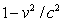the smaller than the surface that contributed the force when the speed of the particle was zero; the profile of the sphere cdt at vdt.

The described situation, where particle Q is only accelerated the force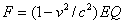, is comparable with someone pushing a pram. In the beginning when the pram has no speed we can contribute all our energy to the acceleration of the pram. When we run behind the pram as fast as we can we cannot accelerate anymore, despite our effort. When the pram runs as fast as we can push all the energy we produce is lost. We cannot accelerate the pram faster than we can run. The same applies to the energy and force around Q. The electric field around Q cannot push Q faster than it is able to transfer itself.

The sphere around Q in figure 11 represents the electric field surrounding Q with radius cdt. The light gray areas present the cone of the polarized ether surrounding Q still able to adjust in time to the movement of Q and therefore still able to induce a force on Q and accelerate the particle further. The dark gray area represents the surface through which the field is still transporting the force from condenser plates to +Q. The particle is therefore only accelerated with a factorof the original force. Now it becomes clear that in ether a charged particle like electron or proton cannot be accelerated above the speed of light. When v=c the electric field is no longer able to induce a force to accelerate Q. The Lorentz-factor becomes zero and therefore the force F also becomes zero as well. The interaction between the electric field of the particle and the electric field of the condenser does not induce an accelerating force anymore.

Summarizing the previous; the force in an electric field on a non-moving particle with charge Q is:

F=QE

When the particle moves in the direction of the force F with speed v the accelerating force that remains is: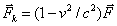Particle Q has not only a charge but also a certain mass M. The acceleration, the increase of speed per second, is proportional to the mass. The bigger the mass the smaller the acceleration will be a given force. A small stone will have a much higher speed when accelerated a catapult than a big stone with the same catapult. In formula:

F=Ma

With M the mass and a the acceleration in the direction of the force. The resulting force Fk of the electric field E on particle Q with mass Mqv gives the equation for acceleration: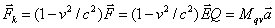When the speed of particle Q is v, and v approaches c one can see in the above formula that the force accelerating Q becomes zero. In an electric field in ether a charged particle can never be accelerated to the speed of light. The lag of the ether, formulated the Lorentzfactor, is responsible for the loss of force compared to a situation where v=0. So the experimental limit c in the ether, vacuum, is not determined now the relativity of time and space, but simply the lag of vacuum to adjust to electrostatic changes. With ether the reason why a charged particle cannot be accelerated above c is that all electrostatic energy/force is lost because of the inability of ether to transfer an electrostatic force/energy to a particle moving with speed c.

The energy contributed a force is the force F multiplied over the distance S where the force is active: W=FS. When v is small (v<<c) the ether will contribute to particle Q all the energy W  accelerating the particle and increasing the speed. Is however the speed v not very small comparable to c than a part of the energy W=FS  is “lost”. “Lost” does not mean that this energy has disappeared.

The original force F has to be split up in a force that still contributes to the acceleration of the particle and a part that is “lost”. “Lost” means here that the energy doesn’t contribute to the speed of the particle.

F=Fk+Fv

Fk is the force still accelerating Q and Fv is the “lost” force. The energy ceded the electric field over a small distance ds can also be split up in a “lost” part Fvds and a part Fkds that is transferred to particle Q and still contributes in the increase of speed:

Fds=Fkds+Fvds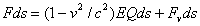The energy “lost” is: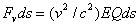As the speed v increases the “lost” energy of the electric field increases. The adaptation of the electric field to the new situation is too slow. Charge Q already moved on. In figure 12 the dark part of the sphere represents the ether too late to contribute to the acceleration. It pictures the energy that is “lost”, because it is not attributing energy to Q. This “lost” energy presents itself as radiation. The lagged electric field will excite the ether behind Q and the ether will release this lagged energy means of electromagnetic waves.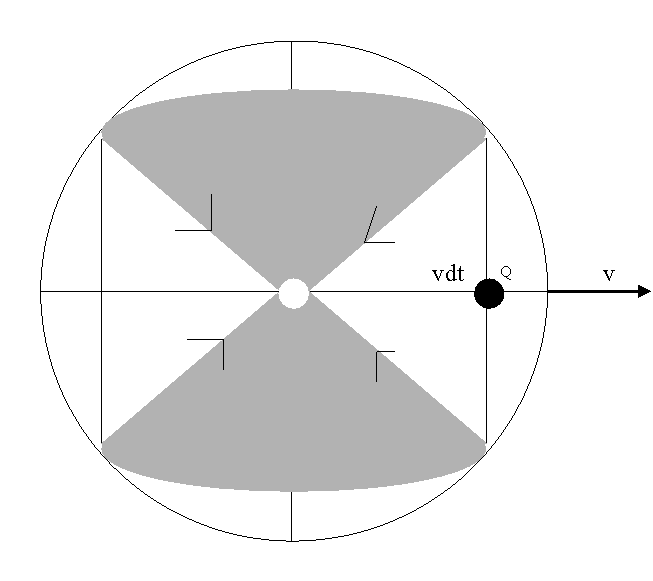Figure 12.  The energy loss during acceleration in an electric field.

When Q moves in a straight line the “lost” energy can catch up with Q because electromagnetic waves travel with the speed of light c and Q can never reach this speed. So the “lost” energy catches up with Q. Particle Q can absorb the radiation and get in a state of electromagnetic vibration. This absorbed electromagnetic energy ceded the electric field however no longer accelerates Q anymore; the “lost” electrostatic energy transformed from electrostatic energy to electromagnetic energy. Particle Q is able to absorb and also emit radiation. When the excitement stage of Q reaches a certain level one can expect that Q will emit this energy in the form of an electromagnetic wave. This characteristic is used to produce synchotron radiation.

The “lost” energy of the field has an impulse of its own. When Q absorbs the electromagnetic radiation the impulse of the radiation will be present in the oscillation of Q. If particle Q’s path is bend the electromagnetic vibration energy of Q is possibly emitted. In physics the radiation emitted during acceleration is known under the name “synchrotron radiation” and is still not accounted for in theory.

The presented perception of the ether is still consistent with observations. We have demonstrated that in ether the force of an electric field on a charge diminishes when the speed increases. To determine the acceleration of particle Q with mass Mqv the equivalence of mass and energy of Einstein has to be accounted for. The mass Mq of a particle at rest, increases to Mqv when the speed is v: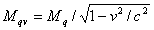Later on we will demonstrate (chapter The Photon), that with ether the mass will increase accordingly to the above formula. In the present perception of science all the energy ceded the electric field contributes to the kinetic energy of the particle. With ether this is not the case. Fv only establishes acceleration while Fk is lost.

Next chapter: The ether again